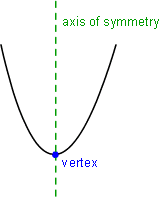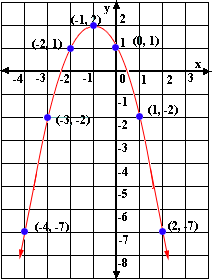Definition Of Parabola

A parabola is the set of all points in a plane that are equidistant from the focus and the directrix of the parabola.The graph of any quadratic equation is a parabola.
All parabolas have an axis of symmetry and the point at which the axis of symmetry intersects the parabola is called the vertex of the parabola and the vertex lies half way between the focus and the directrix.Directrix is a line that is perpendicular to the axis of symmetry of a parabola.
Focus of a parabola lies on the axis of symmetry.

Solved Example on Parabola

Ques: Find the equation of the parabola shown.Choices:

A. y = x3 + 2x + 1
B. y = - x2 + 2x3 + 1
C. y = x2 - 2x + 1
D. y = - x2 - 2x + 1